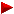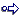HOME plateWON | World!OfNumbersExtraordinary squares and powers(non-palindromic allowed)trianglesquarepentahexaheptaoctanona

Introduction

Palindromic numbers are numbers which read the same fromleft to right (forwards) as from the right to left (backwards)Here are a few random examples : 9, 9559, 4078704, 9876543210123456789

Extraordinary (Palindromic) Squares and Powers

A palindromic chain reaction starting from 1001

10012 = 1002001 and
10020012 = 1004006004001

A palindromic chain reaction starting from 109

109 is a basenumber for the following palindromic triangular 5995.
The sum of the squares of the digits of 5995 is :

52 + 92 + 92 + 52 = 212
and
2122 = 44944

This  palindrome  is also the smallest square containing exactly four digits 4's ! (Sloane's A036511)

SPDS palindromes
by Felice Russo (email) [ July 5, 1999 ]

By solving a problem in Vol. 29 of “Journal of Recreational Mathematics”
Felice Russo stumbled on two interesting palindromic numbers: 121 and 212.
If we take the square of these two palindromes we obtain: 14641 and 44944.
Both these palindromic squares are in SPDS (Square-Partial-Digital-Subsequences).
A number is said to be an element of the set SPDS if it is a square
which can be partitioned into two or more numbers that are also squares.
For example 14641 can be partitioned into the following squares: 1, 4, 64, 4
More interesting the palindrome 44944 that can be partitioned in five squares
that are also single digit palindromes: 4, 4, 9, 4, 4.
By a computer search these are the only palindromes in SPDS up to 3*10^8.
How many SPDS palindromes exist beyond 3*10^8 ?

A response from Alain Bex (email) [ December 28, 1999 ]

"Infinitely many. (if 0 is a square)
every number of the form :

2 ... n zero's ... 1 ... n zero's ... 2 (which is a palindrome)
has a square :

4 ... n zero's ... 4 ... n zero's ... 9 ... n zero's ... 4 ... n zero's ... 4
(which is also a palindrome).
I searched all squares from 0 to the square of 270*10^6 and did not found any others."

Fascinating square of 1003 digits

Calculate the next smallest non trivial square starting with '9'.
This gives the square '961' or 31^2. (900 is excluded as the next square
must be non trivial meaning not ending in zero's)
Repeat this procedure now not with square '9' but with this new square '961'.
After a tenfold iteration we arrive at this 1003-digit square !

To test your programming skills here's a simple assignment :
Calculate the eleventh iteration. Good luck!
```
9616201620200016202000200000001889198463265350032175809769802525931907463586839
53372943202402012699567212284633760835227930246202000200000002000000000000000286
74570609183461343006847306064000000000000000000000000000000000000000000000000000
00000000000165762890459108592015259933448776693776451231520215691182485548960535
13407845648040095751097710143387381095940620098289505535125614470450939160397698
76896979086347754805195901529462026613507501648758045675165710149033232580911054
95275391508140259645504424098617924718273963483282386760738531854344564367702075
18569743231858613025709254857930447199794751022405596837264849453479919042878461
21230650258983014894678321267198660655507316299804535757670938028350277863843458
14818258133214645546046187760712527279785484496736454520815281195847787074116812
26823301300771758521612706290200804538893679740002426955408231457680947810963158
34124835975647700891238330554238156360187301492206636065480004091531578283770622
92595687694293607658268932513211592034772025```
The root of this monstersquare is the next number of 502 digits
``` 3101000100000001000000000000000143372853045917306715034236530320000000000000000
00000000000000000000000000000000000000000000001000000000000000000000000000000000
00000000000000000000000000000000000000000000000000000000000000000000000000000000
00000000000010603496991036628926304444713741834555298799667027754796246140889648
28492192175159864060941684234739538923381863482793853164978801504095322725503902
27748540628752666464970620036253149337706479443351572242228953285666037295270306
10939087202964086953045```

[ October 24, 2003 ]
Jeff Heleen (email) assembled some Ubasic code
and came up with the following program and the eleventh iteration.

```10   input "Input #:";N:if N=0 then end
20   C=1
30   for X=1 to 999
40   A=N*10^X:M=isqrt(A)
50   B=N*10^X+10^X-1:K=isqrt(B)
60   if K-M>0 then print C;M+1;:else 90
70   color 4:print (M+1)^2:color 7:print
80   N=(M+1)^2:C+=1:cancel for:goto 30
90   next
The very large (2006 digit) square is:

96162016202000162020002000000018891984632653500321758097698025259319074635868395/
33729432024020126995672122846337608352279302462020002000000020000000000000002867/
45706091834613430068473060640000000000000000000000000000000000000000000000000000/
00000000001657628904591085920152599334487766937764512315202156911824855489605351/
34078456480400957510977101433873810959406200982895055351256144704509391603976987/
68969790863477548051959015294620266135075016487580456751657101490332325809110549/
52753915081402596455044240986179247182739634832823867607385318543445643677020751/
85697432318586130257092548579304471997947510224055968372648494534799190428784612/
12306502589830148946783212671986606555073162998045357576709380283502778638434581/
48182581332146455460461877607125272797854844967364545208152811958477870741168122/
68233013007717585216127062902008045388936797400024269554082314576809478109631583/
41248359756477008912383305542381563601873014922066360654800040915315782837706229/
25956876942936076582689325132115920347720258914994974518600054221669090816529552/
61437894236214173621326322905481649506236046822896582106849756893167828306680432/
84252106595764741153008678797865772530987719762684436486962268943451632788553907/
31656642177431506759136251659632668095875384355654848275886209152908202640645654/
85309633115453107968421137508354794003355919210333172509687721211355572517254015/
84966273404725097750293678477710394740725438561482888229734954251750517670696829/
31197667454547117994586808831353669444718073268237891569601608146879839980577855/
68498634230572956495503173144474822989944662967196243585206000154855148644388166/
99332772926750077032264526806408733028864379845899337956585494518830134664037042/
60272665189844312896602443928808140300712823333043585779414780090526790082673840/
39874613154649303834964454713467632342245246537690796683760984228012389608036027/
58114886616724551236161055317248176721364665155501095064349159986496584253872772/
51376287320282709627901684380119477746357992201754685746359044847610551198174793/
674225

and its titanic root (with 1003 digits) is:

98062233404099134876441622495550000000000000000000000000000000132221684961658493/
56044245355497113216355576978978796197322817480193272005001656175142188205512607/
86641784654818466088559784060192956988106411704239660892984201575933925927257050/
93722895752000000000000000000000000000000000000000000000000000000000000000000000/
00000000000000000000000000000000000000000000000000000000000000000000000000000000/
00000000000000000000000000000000000000000000000000000000000000000000000000000000/
00000000000000000000002162395262695446493305898867218755368290302794627516422912/
97852058496812878294382635141749116922272185336610080590262350721905475050291580/
25019094606974160494878769448599322323358462223322770851845953571023019388923016/
69998725035652930764159885003020458848439834918776188362960878122551312120192964/
14090175134199456183573744310455126367521842569362771952871094631058349558872104/
94580220340673831425337024896747506901142043562828036442324931521033685432413885/
2469485859733815887082314200288040945972185```
" The only odd thing I noticed was on the step where the number ended in '...6455044'
on your page, my program ended that step with '...645504'.
However, when looking at the following step at the juncture where this step joins it,
the end is given correctly with '...6455044'. And your final step matched my next to
last step. So if someone can verify that I have a correct 11th step...
I couldn't go any further as UBASIC gave me an overflow error. Jeff. "

Three digits only squares

Here is a square composed only of the digits 1, 4 and 9 ! (Sloane's A006716 and A027675)
6480702115891070212 = 419994999149149944149149944191494441
For a more detailed description surf to arithmetic/digits/squares/three.digits.p
An overview of all squares with threedigit combinations is compiled at the following page :
Squares containing at most three distinct digits

There are also alternatives with other threedigit triplets :
7851539123922 = 616466666144464411161664 (Sloane's A027676 and A027677)
2109129782 = 44484284288828484 (Sloane's A027678 and A027679)

Equal Sums of Like Powers

A remarkable collection of Equal Sums of Like Powers is presented by Chen Shuwen (email) from the People's Republic of China.
His website deals with Integer Solutions of the Diophantine System !

Primes whose reversal is a square

E.g. 123373 reversed gives 373321 = 611 x 611 or 6112 !
(See Sloane's On-Line Encyclopedia of Integer Sequences A007488)
Two of them have a palindromic root :
61 reversed is 16 and 16 = 4 x 4
96979 reversed is 97969 and 97969 = 313 x 313
Larger ones are as always more than welcome. Please send them in !

Pandigital squares

Again from the book “Curious and Interesting Numbers” by David Wells, ed. 1988, page 192 and 193 :
320432 = 1026753849
990662 = 9814072356
Two pandigital squares, including the zero.
Note that the basenumber 99066 can be rotated for 180° and remains unchanged !

Squares with identical halves

Squares consisting of two identical 'halves' and that are called "twin" numbers.
36363636364 2 = 13223140496_13223140496

Here is a second one that I (pdg) found by analogeous reasoning (guessing?) on [ July 2, 2001 ]
63636363637 2 = 40495867769_40495867769
Can you discover more of these tautologeous squares ?

Note that 36363636364 + 63636363637 = 100000000001 ... speaking of palindromes !

 Justin Chan's (email)contribution[ July 11, 2008 ] Is a 2n-digit number with identical halves a square number? Note that such number is c(10^n+1) where 10^(n-1) <= c < 10^n. Let 10^n+1 = m*t^2, where t^2 is the largest square dividing 10^n+1. Then c >= m. If t = 1 (10^n+1 is squarefree) then c >= m = 10^n+1, so no such number is square. Otherwise let c = m*b^2, for any b with t/sqrt(10) < b < t. Then c(10^n+1) = (m*b^2)(m*t^2) = (mbt)^2 and 10^(n-1) <= c < 10^n. In other words, there exists a 2n-digit square with identical halves if and only if 10^n+1 is non-squarefree. Example: n = 11 (the least n such that 10^n+1 is not squarefree) 10^11+1 = 100000000001 = (11^2)*23*4093*8779 = 826446281*11^2 So m = 826446281 and t = 11. t/sqrt(10)~3.47 < 4, so let b=4. (b can be anything between 4 and 10 inclusive, for 7 solutions) c = m*b^2 = 13223140496. mbt = 36363636364 Therefore 36363636364^2 = 13223140496_13223140496. Note that mbt + m(t-b)t = m*t^2 = 10^n+1. Hence the 10^n+1_complement of a solution's base is the base of another solution (if t-b is greater than t/sqrt(10)). e.g. 63636363637^2 = 40495867769_40495867769 (t-b = 11-4 = 7) The next n with a solution is n = 21 (t = 7) with 4 solutions (3 <= b <= 6). b = 3: 4285714285714285714292 = 183673469387755102041_183673469387755102041. Other n with solutions: n = 33 (t = 11), n = 39 (t = 13), n = 55 (t = 11), n = 63 (t = 7) In general, there are families of solutions: n = 22k+11 (11|t), n = 42k+21 (7|t), n = 78k+39 (13|t), and so on. Are there any 2n-digit cubes or higher powers with identical halves? Such number requires that 10^n+1 has at most a squarefree part of sqrt(10^n-1). However, the squarefree part of 10^n+1 seems to be very close to 10^n+1 for n>1, if not 10^n+1 itself.

Concatenations of two successive integers

I found four palindromes that when squared constitute a concatenation of two successive integers.
7272 = 528|529
636362 = 40495|40496
45454542 = 2066115|2066116
9090909092 = 826446280|826446281    [ Nov 10, 2003 ]

Squares showing up as substring of their decimal image

There are squares whereby the basenumbers show up as the middle part of these squares :
4954752 = 245495475625
6250002 = 390625000000
9715822 = 943971582724
1776563442 = 31561776563446336
Or when it is just part of, without being in the exact middle :
%N Substring of its square. under A018834
5050252 = 255050250625
1465097172 = 21465097175420089
And when it ends with the number itself :
%N Automorphic numbers n^2 ends with n. under A003226
182128906252 = 3317093849_18212890625
The following superlarge solution is copied from
HAKMEM - NUMBER THEORY, PRIMES, PROBABILITY - item 59
91038909958933800226077437400817871093762 =
8288083126510855871166119716999101717324_9103890995893380022607743740081787109376

From Sloane's OEIS database

One of my favourites from Sloane's table is A007667.
%N The sum of both 2 and of 3 consecutive squares.
I'll give the solutions for the first five from the list :
5 = 12 + 22 = 02 + 12 + 22
365 = 132 + 142 = 102 + 112 + 122
35645 = 1332 + 1342 = 1082 + 1092 + 1102
3492725 = 13212 + 13222 = 10782 + 10792 + 10802
342251285 = 130812 + 130822 = 106802 + 106812 + 106822

More digital silliness or rotating squares
by Bob Hearn (from the SEQFAN mailing list) [ January 10, 2008 ]

This is a cute pair (but still only a pair):

12222222222 = 1493827159950617284
22222222212 = 4938271599506172841

rotating the result by one place.

 Justin Chan's (email)contribution[ July 11, 2008 ] The first property: 1222222222^2 = 1493827159950617284 2222222221^2 = 4938271599506172841 also occurs for 12 and 21, 122 and 221, and so on, since: 22...21^2 = (22...20)(22...22)+1 = 10(2...22)(22...22)+1 = 10(12...22^2-10...00^2)+1 (where ... denotes an equal number of digits left out) Since 12...22^2 has same number of digits as 10...00^2 and begins with 1, the RHS (right hand side of equation) denotes moving the leading 1 to the end.

 Pieter Post's (email)contribution[ September 16, 2017 ] There is another infinite subsequence (Source OEIS sequence A134514) : The cyclic pair (201023, 320102)*k (for k = 2 and 3) and its squares (40410256529, 102465290404)*k^2. The next in the sequence is: (020001000203, 30200010002)*k, (400040009120406041209, 912040604120900040004)*k^2 (for k = 16, 17,..., 33). In general: (0{n}20{2n+1}10{2n+1}20{n}3, 30{n}20{2n+1}10{2n+1}20{n}3)* k, where lower bound of k = 5*10^(n-1)*sqr(10) and upperbound of k = 3{n+1} for n = 0, 1, 2, 3, 4, etc. For example n = 6 gives lower bound k = 1581139 with lower cyclic pair: (316227800000001581139000000031622784743417, 474341731622780000000158113900000003162278) and corresponding squares: (100000021492841000000214928422500007835889744785236492844223926514928486978524835889, 225000078358897447852364928442239265149284869785248358891000000214928410000002149284) And upperbound k = 3333333 with upper cyclic pair: (666666600000003333333000000066666669999999, 999999966666660000000333333300000006666666) and its corresponding squares: (444444355555564444443555555699999993333332111111222222217777778888888766666660000001, 999999933333321111112222222177777788888887666666600000014444443555555644444435555556)

And here's a solution in base 23:

59L2 = 16B964
BL32 = 6416B9
G4B2 = B96416

Exclusionary Squares and Cubes

E.g.:
2038792 = 41566646641
6391722 = 408540845584

Here is an interesting webpage from the collection of Clif Pickover
Clif Pickover's Extreme Challenges in Mathematics and Morals
Exclusionary Squares and Cubes

Here are some corresponding OEIS entries

With no repeating digits in the base numbers
A112321 Least n-digit number such that its square is exclusionary, or 0 if no such number exists. - Lekraj Beedassy
A112322 Exclusionary square associated to corresponding smallest n-digit number (A112321), or 0 if no such number exists. - Lekraj Beedassy and Klaus Brockhaus
A113951 Largest number whose n-th power is exclusionary (or 0 if no such number exists). - Lekraj Beedassy
A113952 Largest exclusionary n-th power (or 0 if no such number exists). - Lekraj Beedassy
A112993 Exclusionary cubes: cubes of the terms in A112994. - Lekraj Beedassy
A112994 Numbers whose cubes are exclusionary: numbers n such that n and n^3 have no digits in common. - Lekraj Beedassy

With repeating digits in the base numbers
A029783 Exclusionary squares: numbers n such that digits of n are not present in n^2. - Patrick De Geest
A029784 Squares such that digits of sqrt(n) are not present in n. - Patrick De Geest
A029785 Digits of n are not present in n^3. - Patrick De Geest
A029786 Cubes such that digits of cube root of n are not present in n. - Patrick De Geest

```

```

[TOP OF PAGE]

Patrick De Geest - Belgium- Short Bio - Some Pictures